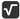## 均分平方根

Mon, 27th January 2020Edit on Github平方根

# 问题起源

2010年3月，sw2wolf求助高德纳书中一道很普通的题目：
$\sqrt{1},\sqrt{2}, \dots, \sqrt{50}$ ( 1 到 50的平方根）分成两组，把每组中的数相加，得出两个数， 然后比较这两个数的差别。

# 算法讨论

winxos认为:
$\sqrt{1}+\sqrt{2}+...+\sqrt{50}=239.0358006035207771$, 一半等于 $119.5179003017603885$,

KeyTo9_Fans不赞同，认为这是0-1背包问题, 表示没有有效算法。

medie2005紧接着提出他的想法, 认为可以对数据进行量化:

$a_{1},a_{2},...,a_{50}$

${b_{1},b_{2},...,b_{k}}$

$b_{1}+b_{2}+...+b_{k}=119517900$

Fans忍无可忍，给出了更加详细的解释:

$\sqrt{1}$,$\sqrt{2}$,$\sqrt{3}$,...,$\sqrt{25}$$25$个数中，取其子集，有$2^{25}$种选取方案。

$0$
$\sqrt{1}$
$\sqrt{2}$
$\sqrt{3}$
$\sqrt{4}$
$\sqrt{5}$
$\sqrt{1}+\sqrt{2}$
$\sqrt{6}$
$\sqrt{7}$
$\sqrt{1}+\sqrt{3}$
$\sqrt{8}$
$\sqrt{1}+\sqrt{4}$
$\sqrt{9}$
$\sqrt{2}+\sqrt{3}$
$\sqrt{10}$
……
（中间省略$33554415$个数）
……
$\sqrt{2}+\sqrt{3}+\sqrt{4}+...+\sqrt{25}$
\sqrt{1}+\sqrt{2}+\sqrt{3v+...+\sqrt{25}

$\sqrt{26}$,$\sqrt{27}$,$\sqrt{28}$,...,$\sqrt{50}$$25$个数中，取其子集，有$2^{25}$种选取方案。

$0$
$\sqrt{26}$
$\sqrt{27}$
$\sqrt{28}$
$\sqrt{29}$
……
（中间省略$33554425$个数）
……
$\sqrt{27}+\sqrt{28}+\sqrt{29}+...+\sqrt{50}$
$\sqrt{26}+\sqrt{27}+\sqrt{28}+...+\sqrt{50}$

liangbch表示还要考虑二分法查找需要的时间和空间复杂度问题.
Fans举例表示可以通过归并的方法降低复杂度，并给出了很具体的例子。

zgg___表示还可以稍微优化一下:

# 结果展示

## 初步结果

wayne首先通过进化的方法给出了一组结果:
{1, 6, 7, 8, 9, 10, 13, 15, 16, 20, 21, 22, 23, 27, 28, 30, 35, 36, 37, 41, 43, 44, 47, 48, 50}
{2, 3, 4, 5, 11, 12, 14, 17, 18, 19, 24, 25, 26, 29, 31, 32, 33, 34, 38, 39, 40, 42, 45, 46, 49}

Title "D. Knuth";
Constant n = 50;
Parameter x(1:n)[0,1,0];
Minimum = True;
Function abs(2*(sum(i=1:n)(sqrt(i)*x[i]))-sum(i=1:n)(sqrt(i)));

{1, 3, 4, 5, 9, 10, 11, 12, 14, 15, 17, 18, 22, 24, 27, 29, 31, 32, 35, 39, 40, 41, 44, 46, 47, 50}
{2, 6, 7, 8, 13, 16, 19, 20, 21, 23, 25, 26, 28, 30, 33, 34, 36, 37, 38, 42, 43, 45, 48, 49}

## 最优结果出现

delta=7.14984e-014:[ 1 4 5 7 8 9 12 13 15 16 20 25 27 30 31 33 37 38 41 43 44 46 47 48 49 ]
delta=7.14984e-014:[ 1 5 7 8 9 12 13 15 20 25 27 30 31 33 36 37 38 41 43 44 46 47 48 49 ]
delta=7.14984e-014:[ 4 5 7 8 9 12 13 15 16 20 27 30 31 33 36 37 38 41 43 44 46 47 48 49 ]
delta=7.14984e-014:[ 5 7 8 12 13 15 16 20 25 27 30 31 33 36 37 38 41 43 44 46 47 48 49 ]
delta=7.14845e-014:[ 1 4 7 8 9 12 13 15 16 25 27 30 31 33 37 38 41 43 44 45 46 47 48 49 ]
delta=7.14845e-014:[ 7 8 12 13 15 16 25 27 30 31 33 36 37 38 41 43 44 45 46 47 48 49 ]
delta=7.14845e-014:[ 4 7 8 9 12 13 15 16 27 30 31 33 36 37 38 41 43 44 45 46 47 48 49 ]
delta=7.14845e-014:[ 1 7 8 9 12 13 15 25 27 30 31 33 36 37 38 41 43 44 45 46 47 48 49 ]

liangbch紧接着给出了他的代码，可以在31秒中找到其中一组结果.

## 速度优化

KeyTo9_Fan_Fans紧接着给出了她的代码（注意不是Fans而是Fans_Fan）和算法介绍:

1 4 7 8 9 12 13 15 16 25 27 30 31 33 37 38 41 43 44 45 46 47 48 49
2 3 5 6 10 11 14 17 18 19 20 21 22 23 24 26 28 29 32 34 35 36 39 40 42 50

Fans大牛的形象是光辉的！伟大的！这种题对于他来说不过是小菜一碟！（嘛，反正事实大家都清楚:D Fans大牛典型的想法灰常好代码灰常那个的，反正手下嘛，用来堆代码做苦力的，有Fans
Fans在就不用亲自披挂上阵了，多好:D）

FansFans现在不知道该说啥，又急着打游戏去，所以说的挺没逻辑的，窘……反正中心思想是：代码是FansFans的，想法是Fans的，嗯。

Fans使用的Fans提供的量化表格竟然和mathe的一字不差。

## 总结

KeyTo9_Fans还对上面代码做了总结性发言
Fans的想法基本上都实现了。

Github